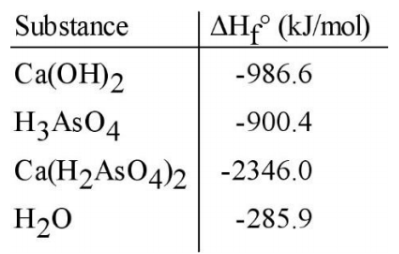# Problem: Given the data in the table on the right, the ΔH°rxn for the reaction:Ca(OH)2 + 2 H3AsO4 → Ca(H2AsO4)2 + 2 H2O  is ___________ kJ.A. -744.9B. -4519C. -4219D. -130.4E. -76.4

###### FREE Expert Solution

We are asked to determine the ΔH°rxn for the reaction

Ca(OH)2 + 2 H3AsO4 → Ca(H2AsO4)2 + 2 H2

We can use the following equation to solve for ΔH˚rxn:

Note that we need to multiply each ΔH˚f by the stoichiometric coefficient since ΔH˚f is in kJ/mol.

Also, note that ΔH˚f for elements in their standard state is 0.

79% (252 ratings)###### Problem Details

Given the data in the table on the right, the ΔH°rxn for the reaction:

Ca(OH)2 + 2 H3AsO4 → Ca(H2AsO4)2 + 2 H2 is ___________ kJ.A. -744.9
B. -4519
C. -4219
D. -130.4
E. -76.4